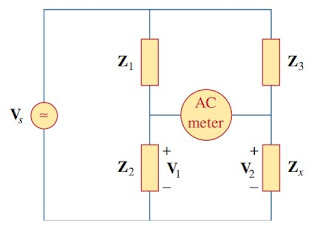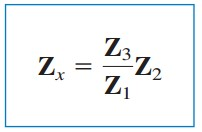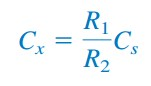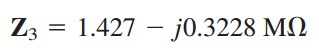omG8HI9LSKFW6lhCj8prTs0Z6lUhdOT9Jhi1Sf4m

# AC Bridge Circuit Formula and Theory of Operation

An ac bridge circuit is one of the simple application of RLC ac circuits along with phase shifter. This bridge circuit is used in measuring the inductance L of an inductor or the capacitance C of a capacitor.

## AC Bridge

It is similar in form to the Wheatstone bridge for measuring an unknown resistance. To measure L and C, however, an ac source is required as well as an ac meter instead of the galvanometer. The ac meter may be a sensitive ac ammeter or voltmeter.

Consider the general ac bridge circuit displayed in Figure.(1).Figure 1. A general ac bridge
The bridge is balanced when no current flows thorugh the meter. This means that V1V2. Applying the voltage division principle,(3)
This is the balanced equation for the ac bridge.

Spesific ac bridges for measuring L and C are drawn in Figure.(2), where Lx and Cx are the unknown inductance and capacitance to be measured while Ls and Cs are a standard inductance and capacitance (the values of which are known to great precision). In each case, two resistors, R1 and R2, are varied until the ac meter reads zero. Then the bridge is balanced. From Equation.(3), we get(5)
Notice that the balancing of the ac bridges in Figure.(2) does not depend on the frequency f of the ac source, since f does not appear in the relationships in Equations.(4) and (5).Figure 2. Spesific ac bridges: (a) for measuring L, (b) for measuring C

## AC Bridge Example

For a better understanding let us review example below :
1. The ac bridge circuit of Figure.(1) balances when Z1 is a 1 kΩ resistor, Z2 is a 4.2 kΩ resistor, Z3 is a parallel combination of a 1.5 MΩ resistor and a 12 pF capacitor, and f = 2kHz. Find: (a) the series components that make up Zx, and (b) the parallel components that make up Zx.
Solution :
From Equation.(3),
(a) Assuming that Zx is made up of series components, we substitute Equations.(1.2) and (1.3) in (1.1) and get(1.4)
Equating the real and imaginary parts yields Rx = 5.993 MΩ and a capacitive reactance
(b) Zx remains the same as in Equation.(1.4) but Rx and Xx are in parallel. Assuming an RC parallel combination,
By equating the real and imaginary parts, we get
Related Posts
SHARE